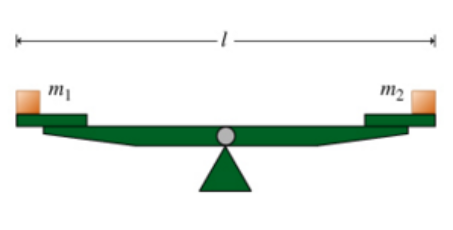# Problem: Learning Goal:To understand and apply the formula τ = Iα to rigid objects rotating about a fixed axis. To find the acceleration a of a particle of mass m, we use Newton's second law. Fnet = ma, where Fnet is the net force acting on the particle.To find the angular acceleration α of a rigid object rotating about a fixed axis, we can use a similar formula τnet = Iα, where τnet = Στ is the net torque acting on the object and I is its moment of inertia.In this problem, you will practice applying this formula to several situations involving angular acceleration In all of these situations, two objects of masses m1 and m2 are attached to a seesaw. The seesaw is made of a bar that has length l and is pivoted so that it is free to rotate in the vertical plane without friction.You are to find the angular acceleration of the seesaw when it is set in motion from the horizontal position. In all cases, assume that m1 &gt; m2, and that counterclockwise is considered the positive rotational direction.Part E. This time, the swing bar of mass mbar is pivoted at a different point, as shown in the figure (Figure 3). Find the magnitude of the angular acceleration α of the swing bar. Be sure to use the absolut value fuction in your answer, since no comparison of m1, m2, and mbar has been made. Express your answer in terms of some or all of the quantities m1, m2, mbar, l, as well as the acceleration due to gravity g.

###### FREE Expert Solution

The total moment of inertia about the hinge is:

$\begin{array}{rcl}\mathbf{I}& \mathbf{=}& {\mathbf{m}}_{\mathbf{1}}{\mathbf{\left(}\frac{\mathbf{l}}{\mathbf{2}}\mathbf{\right)}}^{\mathbf{2}}\mathbf{+}{\mathbf{m}}_{\mathbf{2}}{\mathbf{\left(}\frac{\mathbf{l}}{\mathbf{2}}\mathbf{\right)}}^{\mathbf{2}}\mathbf{+}\frac{\mathbf{1}}{\mathbf{12}}\mathbf{\left(}{\mathbf{m}}_{\mathbf{bar}}{\mathbf{l}}^{\mathbf{2}}\mathbf{\right)}\\ & \mathbf{=}& \frac{{\mathbf{m}}_{\mathbf{1}}{\mathbf{l}}^{\mathbf{2}}}{\mathbf{4}}\mathbf{+}\frac{{\mathbf{m}}_{\mathbf{2}}{\mathbf{l}}^{\mathbf{2}}}{\mathbf{4}}\mathbf{+}\frac{{\mathbf{m}}_{\mathbf{bar}}{\mathbf{l}}^{\mathbf{2}}}{\mathbf{12}}\\ & \mathbf{=}& \frac{\mathbf{3}{\mathbf{m}}_{\mathbf{1}}{\mathbf{l}}^{\mathbf{2}}\mathbf{+}\mathbf{3}{\mathbf{m}}_{\mathbf{2}}{\mathbf{l}}^{\mathbf{2}}\mathbf{+}{\mathbf{m}}_{\mathbf{bar}}{\mathbf{l}}^{\mathbf{2}}}{\mathbf{12}}\\ & \mathbf{=}& \mathbf{\left(}\mathbf{3}{\mathbf{m}}_{\mathbf{1}}\mathbf{+}\mathbf{3}{\mathbf{m}}_{\mathbf{2}}\mathbf{+}{\mathbf{m}}_{\mathbf{bar}}\mathbf{\right)}\mathbf{\left(}\frac{{\mathbf{l}}^{\mathbf{2}}}{\mathbf{12}}\mathbf{\right)}\end{array}$

89% (90 ratings)###### Problem Details

Learning Goal:

To understand and apply the formula τ = Iα to rigid objects rotating about a fixed axis.

To find the acceleration a of a particle of mass m, we use Newton's second law. Fnet = ma, where Fnet is the net force acting on the particle.

To find the angular acceleration α of a rigid object rotating about a fixed axis, we can use a similar formula τnet = Iα, where τnet = Στ is the net torque acting on the object and I is its moment of inertia.

In this problem, you will practice applying this formula to several situations involving angular acceleration In all of these situations, two objects of masses m1 and m2 are attached to a seesaw. The seesaw is made of a bar that has length l and is pivoted so that it is free to rotate in the vertical plane without friction.

You are to find the angular acceleration of the seesaw when it is set in motion from the horizontal position. In all cases, assume that m1 > m2, and that counterclockwise is considered the positive rotational direction.Part E. This time, the swing bar of mass mbar is pivoted at a different point, as shown in the figure (Figure 3). Find the magnitude of the angular acceleration α of the swing bar. Be sure to use the absolut value fuction in your answer, since no comparison of m1, m2, and mbar has been made. Express your answer in terms of some or all of the quantities m1, m2, mbar, l, as well as the acceleration due to gravity g.